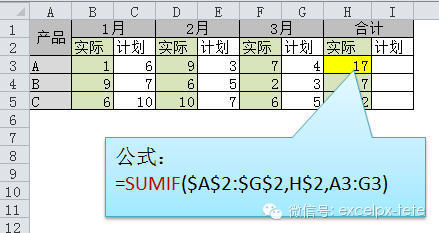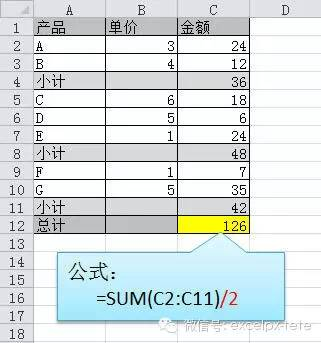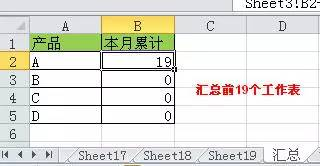# 求和公式excel∑计算简单易学的方法详解说明1、最快数据行公式求和2、行、列最快求和3、多区域最快求和4、最快隔列求和

=SUMIF(\$A\$2:\$G\$2,H\$2,A3:G3)

=SUMPRODUCT((MOD(COLUMN(B3:G3),2)=0)*B3:G3)

{=SUM(VLOOKUP(A3,A3:G3,ROW(1:3)*2,0))} 数组公式5、最快总计求和

=SUM(C2:C11)/26、最快跨多表求和B2单元格输入公式 =SUM('*'9!B2) 后会自动变为：

=SUM(Sheet1:Sheet19!B2)